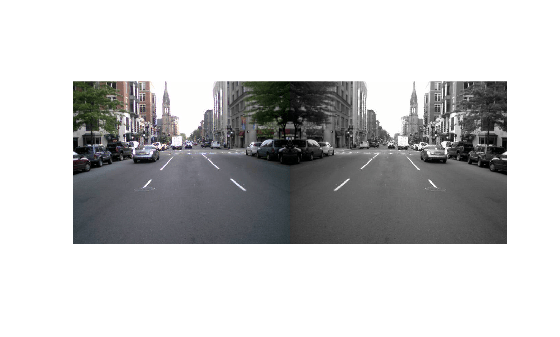# preview

Preview subset of data in datastore

## Syntax

``data = preview(ds)``

## Description

example

````data = preview(ds)` returns a subset of data from datastore `ds` without changing its current position.```

## Examples

collapse all

Create a datastore from the sample file, `airlinesmall.csv`, which contains tabular data.

```ds = tabularTextDatastore('airlinesmall.csv','TreatAsMissing','NA',... 'MissingValue',0);```

Modify the `SelectedVariableNames` property to specify the variables of interest.

`ds.SelectedVariableNames = {'DepTime','ArrTime','ActualElapsedTime'};`

Preview the data for the selected variables.

`data = preview(ds)`
```data=8×3 table DepTime ArrTime ActualElapsedTime _______ _______ _________________ 642 735 53 1021 1124 63 2055 2218 83 1332 1431 59 629 746 77 1446 1547 61 928 1052 84 859 1134 155 ```

Create a datastore from the sample file, `mapredout.mat`, which is the output file of the `mapreduce` function.

`ds = datastore('mapredout.mat');`

Preview the data in the datastore.

`data = preview(ds)`
```data=1×2 table Key Value ______ _________ {'AA'} {} ```

Create a datastore that maintains parity between the pair of images of the underlying datastores. For instance, create two separate image datastores, and then create a combined datastore representing the two underlying datastores.

Create an image datastore `imds1` representing a collection of three images.

`imds1 = imageDatastore({'street1.jpg','street2.jpg','peppers.png'}); `

Create a second datastore `imds2` by transforming the images of `imds1` to grayscale and then reflecting the images horizontally.

`imds2 = transform(imds1,@(x) fliplr(im2gray(x)));`

Create a combined datastore from `imds1` and `imds2`.

`imdsCombined = combine(imds1,imds2);`

Preview the data in the combined datastore. The output is a 1-by-2 cell array. The two columns represent the first subset of data from the two underlying datastores `imds1` and `imds2`, respectively.

`dataOut = preview(imdsCombined)`
```dataOut=1×2 cell array {480x640x3 uint8} {480x640 uint8} ```

Display the previewed data as a pair of tiled images.

```tile = imtile(dataOut); imshow(tile)```## Input Arguments

collapse all

Input datastore. You can use these datastores as input to the `preview` method.

## Output Arguments

collapse all

Subset of data, returned as a table or an array depending on the type of `ds`.

Type of DatastoreData type of `data`Description
`TabularTextDatastore` and `SpreadsheetDatastore`TableTable with variables specified by the `SelectedVariableNames` property. The table contains at most eight rows.
`ImageDatastore`Integer array

Array of integers corresponding to the first image. The dimensions of the integer array depend on the type of image:

• For grayscale images, `data` is m-by-n.

• For truecolor images, `data` is m-by-n-by-3.

• For CMYK Tiff images, `data` is m-by-n-by-4.

The `preview` function supports all image types supported by the `imread` function. For more information on the supported image types, see `imread`.

`KeyValueDatastore`TableTable with the variables `Key` and `Value`.
`FileDatastore`TableTable containing the output returned by the read function, specified by the `'ReadFcn'` parameter in the `fileDatastore` function.
`TransformedDatastore`VariesThe output is the same as the output returned by the underlying datastore specified by the `UnderlyingDatastores` property. For example, if the underlying datastore is an image datastore with a `ReadSize` property value of 1, then `data` is returned as a integer array.
`CombinedDatastore`Cell arrayEach element of the cell array contains the output returned by the corresponding underlying datastore specified by the `UnderlyingDatastores` property.
`SequentialDatastore`VariesThe output is a small amount of data from the first nonempty underlying datastore. If all underlying datastores are empty, output is the empty type based on the first underlying datastore. If there are no underlying datastores, output is an empty double.

## Version History

Introduced in R2014b